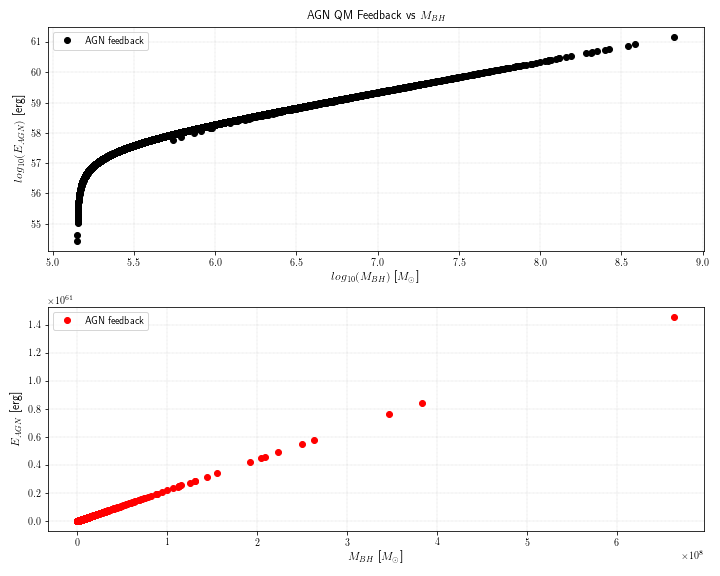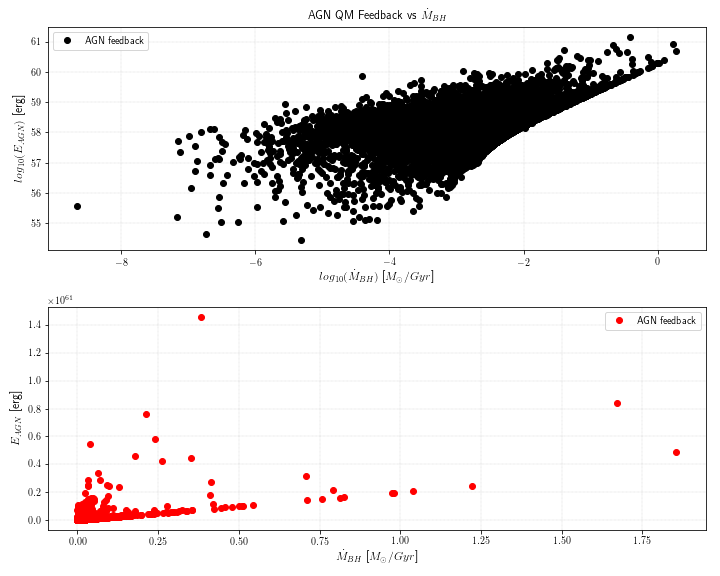### AGN Feedback and Stellar FeedbackFlaminia Fortuni
• 31 Jul '18

Hello everyone, I'm trying to study feedback mechanisms responsible for quenching the star formation activity in galaxies (SubHalo), both AGN and stellar feedback . I'm using datas from both Snapshot files (PartType5) and from SubFind files. I have a couple of questions:

1) For AGN feedback, I'm using information stored in PartType5. The field "BH CumEgy Injection QM" is described as "Cumulative amount of thermal AGN feedback energy injected into surrounding gas in the quasar mode" and I think it is the information that I need to estimate AGN feedback, but it is given in units of M_sun * (ckpc/Gyr)^2. Is it right to convert it in erg and considerate it as the AGN feedback?

2) For Stellar feedback . I don't see any Fields for stellar feedback in PartType4. So I think I can use SubHaloWindMass information from SubFind files, but it's not enough to estimate stellar feedback. How can I estimate it?

Thanks for helping me. FlaminiaDylan Nelson
• 16 Aug '18

Hi Flaminia,

Estimating either type of feedback energy is fairly difficult, unforunately.

For AGN, yes you can use this field, but it gives you only information about the "quasar mode". The "radio mode" is at least as important, and possibly more so, but there is no direct tracking of this in Illustris. You can estimate it approximately by looking at the equations for energy injection by black holes, which depend e.g. on their gas accretion rates, and this stored data from the snapshots to reconstruct the history of energy injection by each BH particle.

Similarly, for stellar feedback, the best approach would be to use the SFR of the galaxy at each point back in time, then use the equations (from the methods papers) which convert this value into the amount of energy used to generate winds. This will be approximate. The SubhaloWindMass field isn't going to be useful.Flaminia Fortuni
• 1
• 22 Aug '18

Thanks a lot. Just other two questions:

Is there an empirical relation between quasar-mode and radio-mode AGN feedback? It would be useful to estimate radio-mode feedback from quasar-mode, just a raw estimation I mean.

About stellar feedback, can you suggest the method paper in which they describe the conversion between SFR and energy from winds? I'm searching for it, but there's so much material to check. I found something in Vogelsberger 2013, eq. 16, but it's not the conversion you talked about. I would be grateful- thanks.Dylan Nelson
• 22 Aug '18

Hi Flaminia,

For the BH feedback, you can of course take the ratio of the Edot equations corresponding to the two modes, to determine the ratio of the energy which would have been injected at a given Mdot. But, this would be just at one instant in time. I think you mean, integrated over the entire lifetime of the BH (e.g. to z=0). This depends on the precise accretion history of the BH (e.g. Figure 7 of Nelson+ 2018 for the new TNG sims), and unfortunately isn't tracked in Illustris.

As an estimate, you could try to work with `BH_CumMassGrowth_QM`. If you subtract this from the actual BH mass at z=0, then you should be left with two terms: mergers, and radio-mode. If you subtract out the merger-mass growth (e.g. using the BH details/mergers supplementary catalogs), then you are left with the total mass accumulated in the radio-mode. These total masses, combined with the efficiency factors, should give the total energy injection per mode.

For the stellar feedback, look at Eqns 3 and 5 of Pillepich+ (2018), this is the TNG methods paper. Also useful to look at Section 2.3 of Weinberger+ (2018). Note that both of these are for the TNG model, but can be adapted to original Illustris by just setting the parameters to the original values (e.g. tau_w=0, f_w,Z=0).Flaminia Fortuni
• 28 Aug '18

Thank you for your suggestions. I found a relation that I didn't expect between the cumulative amount of thermal AGN feedback energy and the BH mass at z=4 (snapshot 54). Is there a physical explanation? I'd rather expect this relation between thermal AGN feedback energy and BH accretion rate (Weinberg+2018).Dylan Nelson
• 28 Aug '18

Hi Flaminia,

The relation between instantaneous E_AGN (thermal) and Mdot is given by Eqn. 7 of Weinberger+ 2017, i.e. `E = eps_f,high * eps_r * Mdot * c^2` where `eps_r=0.2` and `eps_f,high = 0.05` (for Illustris). If you integrate this over any `dt`, then you can see that the cumulative E_AGN should be related directly to the final M_BH.Flaminia Fortuni
• 1 Sep '18

so the BH_CumEgyInjection_QM (cumulative amount of thermal AGN feedback energy injected into surrounding gas in the quasar mode) given in snapshot files is integrated over the history of the object or over an arbitrary dt (e.g. dt between the current snapshot and the previous one)?Dylan Nelson
• 2 Sep '18

Hi Flaminia,

This is integrated over the entire lifetime of the blackhole, since formation. I'll clarify this in the docs, thanks!

You can find a given BH at each snapshot back in time, and take the difference of this field between each adjacent snapshot pair normalized by the dt between those two snapshots, to calculate an 'instantaneous' (time-averaged over ~150 Myr) value.

• Page 1 of 1